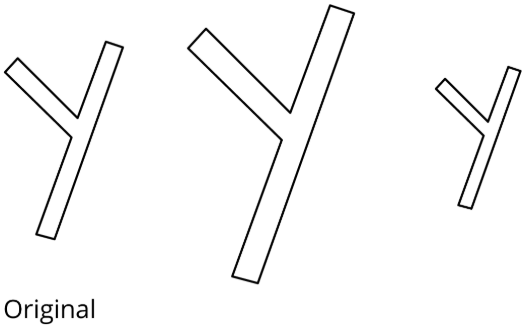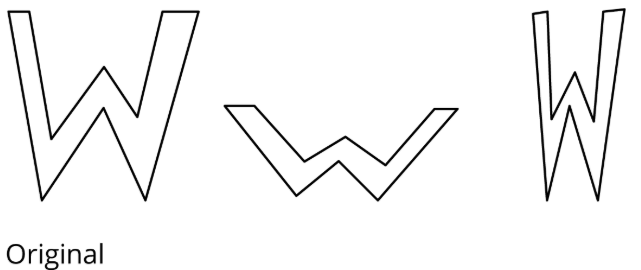# 1.1.1: What are Scaled Copies?

•• Illustrative Mathematics
• OpenUp Resources
$$\newcommand{\vecs}{\overset { \rightharpoonup} {\mathbf{#1}} }$$ $$\newcommand{\vecd}{\overset{-\!-\!\rightharpoonup}{\vphantom{a}\smash {#1}}}$$$$\newcommand{\id}{\mathrm{id}}$$ $$\newcommand{\Span}{\mathrm{span}}$$ $$\newcommand{\kernel}{\mathrm{null}\,}$$ $$\newcommand{\range}{\mathrm{range}\,}$$ $$\newcommand{\RealPart}{\mathrm{Re}}$$ $$\newcommand{\ImaginaryPart}{\mathrm{Im}}$$ $$\newcommand{\Argument}{\mathrm{Arg}}$$ $$\newcommand{\norm}{\| #1 \|}$$ $$\newcommand{\inner}{\langle #1, #2 \rangle}$$ $$\newcommand{\Span}{\mathrm{span}}$$ $$\newcommand{\id}{\mathrm{id}}$$ $$\newcommand{\Span}{\mathrm{span}}$$ $$\newcommand{\kernel}{\mathrm{null}\,}$$ $$\newcommand{\range}{\mathrm{range}\,}$$ $$\newcommand{\RealPart}{\mathrm{Re}}$$ $$\newcommand{\ImaginaryPart}{\mathrm{Im}}$$ $$\newcommand{\Argument}{\mathrm{Arg}}$$ $$\newcommand{\norm}{\| #1 \|}$$ $$\newcommand{\inner}{\langle #1, #2 \rangle}$$ $$\newcommand{\Span}{\mathrm{span}}$$$$\newcommand{\AA}{\unicode[.8,0]{x212B}}$$

## Lesson

Let's explore scaled copies.

Exercise $$\PageIndex{1}$$: Printing Portraits

Here is a portrait of a student. Move the slider under each image, A–E, to see it change.

1. How is each one the same as or different from the original portrait of the student?
2. Some of the sliders make scaled copies of the original portrait. Which ones do you think are scaled copies? Explain your reasoning.
3. What do you think “scaled copy” means?

Exercise $$\PageIndex{2}$$: Scaling F

Here is an original drawing of the letter F and some other drawings.Figure $$\PageIndex{1}$$
1. Identify all the drawings that are scaled copies of the original letter F drawing. Explain how you know.
2. Examine all the scaled copies more closely, specifically, the lengths of each part of the letter F. How do they compare to the original? What do you notice?
3. On the grid, draw a different scaled copy of the original letter F.

Exercise $$\PageIndex{3}$$: Pairs of Scaled Polygons

Your teacher will give you a set of cards that have polygons drawn on a grid. Mix up the cards and place them all face up.

1. Take turns with your partner to match a pair of polygons that are scaled copies of one another.
1. For each match you find, explain to your partner how you know it’s a match.
2. For each match your partner finds, listen carefully to their explanation, and if you disagree, explain your thinking.
2. When you agree on all of the matches, check your answers with the answer key. If there are any errors, discuss why and revise your matches.
3. Select one pair of polygons to examine further. Use the grid below to produce both polygons. Explain or show how you know that one polygon is a scaled copy of the other.

Are you ready for more?

Is it possible to draw a polygon that is a scaled copy of both Polygon A and Polygon B? Either draw such a polygon, or explain how you know this is impossible.

### Summary

What is a scaled copy of a figure? Let’s look at some examples.

The second and third drawings are both scaled copies of the original Y.Figure $$\PageIndex{2}$$

However, here, the second and third drawings are not scaled copies of the original W.Figure $$\PageIndex{3}$$

The second drawing is spread out (wider and shorter). The third drawing is squished in (narrower, but the same height).

We will learn more about what it means for one figure to be a scaled copy of another in upcoming lessons.

### Glossary Entries

Definition: Scaled Copy

A scaled copy is a copy of an figure where every length in the original figure is multiplied by the same number.

For example, triangle $$DEF$$ is a scaled copy of triangle $$ABC$$. Each side length on triangle $$ABC$$ was multiplied by 1.5 to get the corresponding side length on triangle $$DEF$$.Figure $$\PageIndex{4}$$

## Practice

Exercise $$\PageIndex{4}$$

Here is a figure that looks like the letter A, along with several other figures. Which figures are scaled copies of the original A? Explain how you know.Figure $$\PageIndex{5}$$

Exercise $$\PageIndex{5}$$

Tyler says that Figure B is a scaled copy of Figure A because all of the peaks are half as tall.

Do you agree with Tyler? Explain your reasoning.Figure $$\PageIndex{6}$$: Two 12 sided figures in the shape of a W are labeled A and B. Both figures are composed of vertical and horizontal line segments that meet at a right angle. In figure A, the height is labeled 4 centimeters and in figure B, the height is labeled 2 centimeters. The width of both figures, appear to be the same, and is not labeled.

Exercise $$\PageIndex{6}$$

Here is a picture of the Rose Bowl Stadium in Pasadena, CA.Figure $$\PageIndex{7}$$: Rose Bowl aerial, by NASA (http://worldwind.arc.nasa.gov/). Public Domain. Wikimedia Commons. Source.

Here are some copies of the picture. Select all the pictures that are scaled copies of the original picture.Figure $$\PageIndex{8}$$

Exercise $$\PageIndex{7}$$

Complete each equation with a number that makes it true.

1. $$5\cdot \underline{\qquad}=15$$
2. $$4\cdot\underline{\qquad}=32$$
3. $$6\cdot\underline{\qquad}=9$$
4. $$12\cdot\underline{\qquad}=3$$

This page titled 1.1.1: What are Scaled Copies? is shared under a CC BY license and was authored, remixed, and/or curated by Illustrative Mathematics.